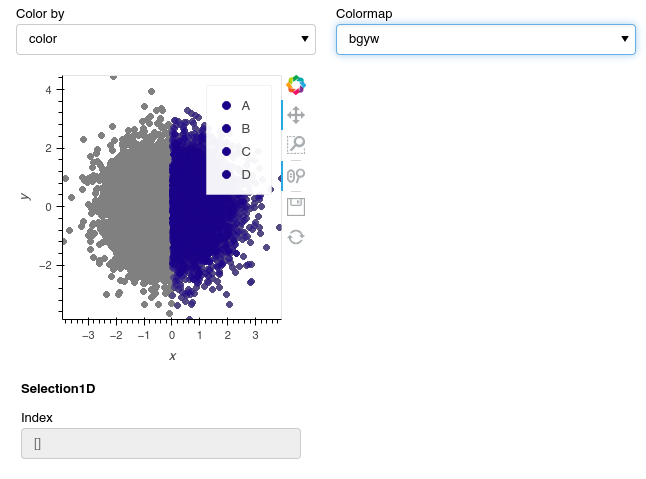# Issues with datashading interactive plot

Having a tough time moving my functional dashboard over into a version that can handle the hundreds of thousands of datapoints I’d like to be able to handle using datashader. As a minimum reproducible example I’m just focusing on the point plot that is the main component of my dashboard. These are roughly my requirements:

1. Selection streaming
2. show selected points as colored, nonselected points as grey (ideally with an adjustable alpha but I’ll accept if that’s not possible)
3. Use of a widget to control which column is used to color the point plot. Some of these are categorical, and some are numerical, which creates some issues due to a bug, so I am using pn.bind as a workaround (DynamicMap Issue: AttributeError: unexpected attribute 'factors' to LinearColorMapper · Issue #5591 · holoviz/holoviews · GitHub)
4. legend/colorbar

Been having a lot of trouble getting something working but now I have this:

``````import numpy as np
import pandas as pd
import hvplot.pandas
import holoviews as hv
from holoviews import opts
import panel as pn
from colorcet import palette
from holoviews.streams import Selection1D

# Create a sample dataset
np.random.seed(42)
data = pd.DataFrame({
'x': np.random.randn(10000),
'y': np.random.randn(10000),
'color': np.random.choice(['A', 'B', 'C', 'D'], size=10000)
})

# Define the points function
def points(dataset, colorby=None):
return dataset.data.hvplot(kind='points',x='x', y='y', legend=False, tools=['box_select'])

# Create widgets
color_by_widget = pn.widgets.Select(name='Color by', options=['color'], value='color')
cmap_widget = pn.widgets.Select(name='Colormap', options=['kbc', 'fire', 'bgy', 'bgyw', 'bmy', 'gray', 'rainbow4'], value='kbc')

# Create the initial static_points plot
static_points = hv.Dataset(data).apply(points, colorby=color_by_widget)

# Create Selection1D stream
index_stream = Selection1D(source=static_points)

# Create a filtered dataset (use your own filter criteria)
def filter_ds(dataset, x):
df = dataset.data
df = df.loc[df['x'] > x]
return hv.Dataset(df)
filtered_data = hv.Dataset(data).apply(filter_ds, x=0)

# Define the points_bind function
def points_bind(data, static_points, color_by_column, cmap):
static_points = static_points.opts(opts.Points(color='gray', size=5))

cmap_dict = {cat: color for cat, color in zip(data[color_by_column].unique(), palette[cmap])}

dynamic_points = data.hvplot.points(x='x', y='y', c=color_by_column, cmap=cmap_dict, colorbar=True, legend=False, size=5)

# Create the interactive plot
interactive_plot = pn.bind(points_bind, filtered_data.data, static_points, color_by_widget, cmap_widget)

# Create the dashboard
dashboard = pn.Column(
pn.Row(color_by_widget, cmap_widget),
interactive_plot,
index_stream.param
)

dashboard.servable()
``````

Which is as far as I’ve been able to get without completely losing my mind. This is what the result looks like:It’s a bit buggy but the biggest issues right now is that everything is just being given the same color, and I don’t know how to stream from this. The way I was streaming before was by using `static_points` for selection streaming and then combining that with dynamic points within the bind function, but I had to switch away from that approach to get the datashade coloring to work. I’d like to return to using the `points` function applied to a hv.dataset within the pn.bind function if that’s possible. Any advice would be greatly appreciated!